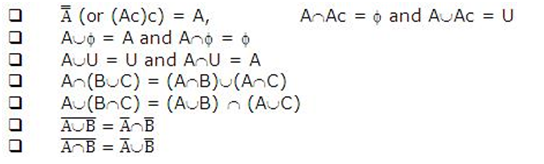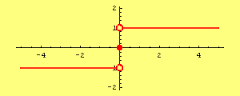Click to Chat

1800-1023-196

+91-120-4616500

CART 0

• 0

MY CART (5)

Use Coupon: CART20 and get 20% off on all online Study Material

ITEM
DETAILS
MRP
DISCOUNT
FINAL PRICE
Total Price: Rs.

There are no items in this cart.
Continue Shopping• Complete JEE Main/Advanced Course and Test Series
• OFFERED PRICE: Rs. 15,900
• View Details

```Revision Notes on Sets, Relations, Functions & Logarithm

Each element in a set is unique.

The elements of a set may be discrete or continuous.

A set may contain no element at all, finite number of elements or infinite number of elements.

If A and B are two sets, then the following results hold true:Relation is a linear operation which establishes relationship between the elements of two sets according to some definite rule of relationship.

If R is a relation from a set A to set B, then the set of all first components or coordinates of the ordered pairs is called the domain of R, while the set of all second components or coordinates of the ordered pairs is called as range of relation.

If R is a relation defined from A to B, then R-1 is a relation defined from B to A as R-1 = {(b,a)| (a, b) ∈ R}.

There can be various types of relations like:

Identity relation

Reflexive relation

Symmetric relation

Transitive relation

Equivalence relation

A relation defined on a set A is said to be an identity relation if every element of A is related to itself and only itself.

A relation such that (a, b) ∈ R then (a, a) ∈ R is reflexive. However, if there is a single ordered pair (a, b) ∈ R, such that (a, a) does not belong to R, then R is not reflexive.

A relation defined on a set is said to be symmetric if a R b ⇒ b R a.

A relation defined on a set A is said to be transitive if a R b and b R c then a R c.

A relation which is reflexive, symmetric as well as transitive is said to be an equivalence relation.

A relation becomes a function iff:

To each a ∈ A, there exists a unique 'b' ∈ B such that (a, b) ∈ f.

(a1, b1) ∈ f and (a1, b2) ∈ f ⇒ b1 = b2.

Let f : A → B, then the domain and codomain of f are defined as

Domain of f = {a | a ∈ A, (a, f(a)) ∈ f}

Range of f = {f(a) | a ∈ A, f(a) ∈ B, (a, f(a)) ∈ f}

Basic Properties of |x|:

| |x| | = |x|

|x| > a ⇒ x < a or x < -a if a ∈ R+ and x ∈ R if a ∈ R-

|x| < a ⇒ -a < x <a if a ∈ R+ and no solution if a ∈ R- ∪ {0}

|x + y| < |x| + |y|

|x - y| > |x| - |y|

The last two properties can be put in one compact form namely,

|x| - |y| < |x + y| < |x| + |y|

|xy| = |x| |y|

|x/y| = |x/y|, y ≠ 0

For ‘y’ to be an algebraic function of x it should satisfy an algebraic expression of the formP0(x)yn + P1(x)yn-1 + ….. + Pn-1(x)y + Pn(x) = 0

All polynomial functions are algebraic but every algebraic function need not be a polynomial function.

Signum Function:

A function y = f(x) = Sgn (x) is defined as follows:

y = f(x) = 1 for x > 0

= 0 for x = 0

= -1 for x < 0Properties of greatest integer function:

[x] ≤ x < [x] + 1

x-1 < [x] ≤ x

[x+m] = [x] + m  if m is an integer

[x] + [y] ≤ [x + y] ≤ [x] + [y] + 1

[x] + [-x] = 0 if x is an integer

= -1 otherwise

Range of y = f(x) is the collection of all outputs corresponding to each real number of the domain. To find the range of a function:

Find the domain of the function y = f(x) first.

If domain is a set having only finite number of points, then range is the set of corresponding f(x) values.

If domain of y = f(x) is R or R- {some finite points}, then express x in terms of y. from this, find y for x to be defined or form an equation in terms of x and apply the condition for real roots.

Two functions ‘f’ and ‘g’ are identical if and only if they satisfy the following conditions:

Domain of f = Domain of g

Range of f = Range of g

f(x) = g(x) ∀x belonging to the common domain

If a function is entirely increasing or decreasing in some entire domain, then the function is one-to one.

If a line parallel to the x-axis cuts the graph of the function at a single point, then the function is one-to-one.

If a continuous function has at least one local maximum or local minimum, then the function is many-one.

A function can either be one-one or many-one but not both.

If range of a function f(x) = codomain of f(x), then the function f(x) is onto.

A polynomial function of even degree is always an into function.

A function can only be one of these four types:

One-one onto (injective and surjective)

One –one into (injective but not surjective)

Many-one onto (surjective but not injective)

Many-one into (neither injective nor surjective)

A continuous function which is always increasing or decreasing in the whole domain is one-one.

A function is one to one iff a horizontal line intersects its graph at most once.

If the range and co-domain for a function are same then it is onto.

If a set A contains ‘n’ distinct elements then the number of possible distinct functions from A to A is nn and out of these n! are one-one.

The range of a constant function is always a singleton.

A constant function may be one-one, many-one, onto or into.

If ‘f’ and ‘g’ are real valued functions with domains as A and B respectively, then both f and g are defined in A∩B.

The domain of f + g, f-g and f.g is also A ∩ B.

The domain of (f/g) is {x | x ∈A ∩ Bs.t. g(x) ≠ 0}.

For the product ‘gof’ of two functions f and g, the range of f must be a subset of the domain of g.

The composite of functions is not commutative, i.e. gof ≠ fog.

The composite of functions is associative i.e. if f, g and h are three functions such that fo(goh) = (fog)oh.

The composite of two bijections is a bijection i.e. if f and g are two bijections such that gof is defined then gof is also a bijection.

The inverse of a bijection is unique.

If f: A→ B is a bijection, and g:B→ A is its inverse, then fog = IB and gof = IA, where IA and IB are the identities of A and B respectively.

The graphs of f and g in this case are the mirror images of each other in the line y = x.

The inverse of a bijection is also a bijection.

If f and g are two bijections such that f: A→ B is a bijection, and g: B→ C, then the inverse of gof also exists and (fog)-1 = f -1og-1

The function is said to be even if f(x)-f(-x) = 0 and the function is said to be odd if f(x) + f(-x) = 0

A function may neither be even nor odd.

Inverse of an even function is not defined.

Every even function is symmetric about the y-axis and every odd function is symmetric about origin.

It is possible to express every function as the sum of an even and odd function i.e.

f(x) = [{f(x) + f(-x)}/2 + {f(x)-f(-x)}/2], where the first part is an even function and the second part is the odd function.

If function f and g are both even or both odd then the function f.g will be even but if either of them is odd then f.g will be odd.

The only function which is defined on the entire number line and which is even as well as od at the same time is f(x) = 0.

The inverse of a periodic function does not exist.

Every constant function is always periodic but with no fundamental period.

If a function f(x) has period ‘T’ and the function g(x) also has the same period, then it is not necessary that the function f(x) + g(x) will also have ‘T’ as its period.

If the function f has ‘p’ as its period then 1/f(x) and √f(x) also have p as the period.

If f(x) has period ‘T’ then f(ax+b) has a period T/a (a > 0).

Logarithm of a number to some base is the exponent by which the base must be raised in order to get that number.

Logarithm of zero does not exist and logarithm of negative reals is not defined in the system of real numbers.

is an identity for all N > 0 and a > , a ≠ 1.

The basic definition of logarithm yields the following results:

logNN = 1

log1/N N = -1

loga1 = 0

a logan =  n is an identity for all N > 0 and a > 0

Whenever the number and base are on the same side of unity then the logarithm of that number to the base is positive, but if the number and base are located on different side of unity, then the logarithm of that number to the base is negative.

Principal properties of logarithm:

If m, n are arbitrary positive real numbers where a > 0, a ≠ 1

logam + logan = logamn

loga m/n = logam - logan

logamx = x logam

logamx = x logam

logax m = 1/x logam

Base changing theorem: It states that the quotient of the logarithm of two numbers is independent of their common base i.e.

logca / logcb = logba

logba = 1/ logab

(logba).(logcb).(logdc) = logd a

alogbc = c logba

log10N is referred to as common logarithm and logeN is called the natural logarithm.

The common logarithm of a number consists of two parts, integral and fractional, of which the integral part may be zero or an integer (positive or negative) while the fractional part, a decimal less than 1 is always positive.

log x2n = 2n log |x|, where n ∈ I.

Note:

Equations of the form [a(x)]b(x) = [a(x)]c(x) with the set of permissible values defined by the condition a(x) > 0, can be reduced to the equivalent equation

b(x) logd[a(x)] = c(x) logd[a(x)] by taking logarithm of both sides. This equation hence is equivalent to the two equations logd[a(x)] = 0, b(x) = c(x).
```### Course Features

• 728 Video Lectures
• Revision Notes
• Previous Year Papers
• Mind Map
• Study Planner
• NCERT Solutions
• Discussion Forum
• Test paper with Video Solution